Science, Maths & Technology

### Become an OU student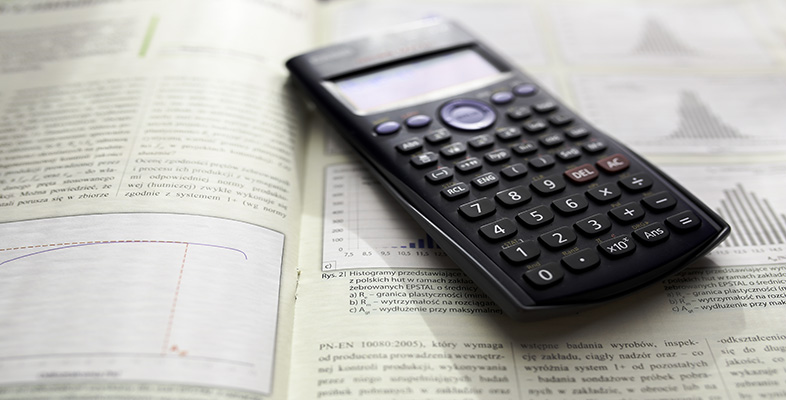Using a scientific calculator

Start this free course now. Just create an account and sign in. Enrol and complete the course for a free statement of participation or digital badge if available.

# 4.2 Using the calculator memory

A variation on the above method is to break the calculation into two parts, and use the memory functions of the calculator to store the result of the first part. The calculator memory is particularly useful when you want to calculate the values of several expressions that have a common part. This common part need be entered only once and its value reused several times subsequently. For example, rewriting the formula for the volume of wood contained in a log as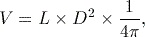we can see that no matter what the values of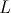and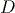, the formula always requires the value of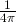. If we wished to calculate the volume of wood contained in several different logs, it might be efficient to calculate the value ofonce, store it in memory and reuse this value in the subsequent calculations.

The calculator has several different memories. We shall first consider the ‘M’ memory, which is accessed using the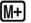key (and its associated functions) at the bottom right-hand corner of the function key area.

Before using the calculator memory, it is good practice to always clear any previous data stored in the calculator using the key sequence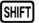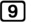(CLR)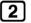(Memory)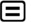(Yes)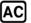.

Note that this clears all the calculator memories.

To store the result of an expression just calculated (i.e. an answer displayed in the output area of the calculator screen) in the ‘M’ calculator memory, use the key sequence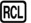(STO)(M). Here we are using the second function of the(or recall) button, which is called ‘STO’ (or store). After selecting the store function, we need to tell the calculator which memory the value is to be stored in. These memories are labelled in red on some of the calculator keys, and the ‘M’ memory is obtained by pressing thekey. We could read the key sequence as ‘store the current result into the M memory’.

Once(or(STO)) has been pressed, the display indicator RCL (or STO) is shown on the display to indicate that the calculator is waiting to know which memory to recall (store) the value from (in).

To display the current contents of the ‘M’ memory, press(M). The value stored in memory can also be used as part of a subsequent calculation by inserting the ‘letter’ M into the appropriate point of the expression using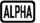(M). For example, to find the square of the value currently stored in the ‘M’ memory,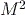, we can use the key sequence(M)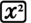.

When there is a value stored in the ‘M’ memory, the display indicator M is shown at the top of the display.

## Activity 12 Using memory

Store the value ofin the ‘M’ memory of the calculator and then use this stored value to evaluate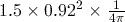to 3 significant figures.

The value of(which equals 0.0796 to 3 significant figures) can be calculated using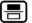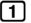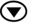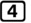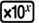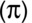and stored in the ‘M’ memory using the key sequence(STO)(M).
This value can then be used to find the final result using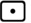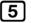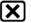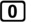(M), which gives 0.101 to 3 significant figures, as expected.